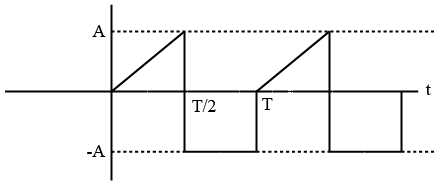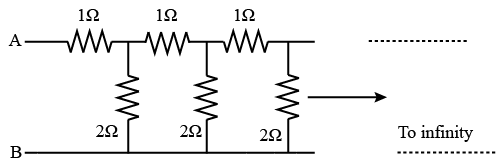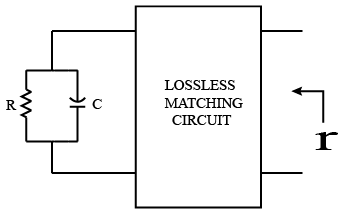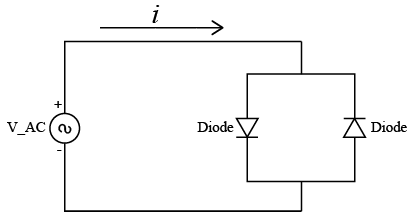# ISRO Scientist or Engineer Electronics 2012

Instructions

For the following questions answer them individually

Question 11

# For Butterworth & Chebyshevfilters, the correct statementis :Question 12

# In a feedback series regulator circuit, the output voltage is regulated by controlling thQuestion 13

# In a fullwave rectifier circuit with centre tap transformer, if voltage between one end of secondary winding and centre tap is 300V peak, then PIV (peak inverse voltage)isQuestion 14

# The rms value of the periodic waveform as shown in Figure isQuestion 15

# The approximate equivalent resistance between terminals A and B for the following infinite ladder network comprising of 1Ω &2Ω resistors is:Question 16

# If the step response to the input step amplitude of 1 V is given by $$V_{o}(t)=(1-e^{\frac{-t}{RC}})$$ the network can be represented by:Question 17

# For a parallel RC circuit as shown in figure, R = 20 QN & C = 0.9 vF, which is to be matched to 50 Q over a bandwidth,$$\int_{0}^{\infty} \ln \frac{1}{\mid r \mid} dw \leq \frac{\pi}{RC}$$The circuit has to be matched over 6 GHz to 18 GHz, What is the  very best r that can be achieved:Question 18

# In this circuit, V is a sinusoid input with freq f, current i contains:Question 19

# The effect of phase error of local oscillator on spectral components in’ coherent detection of SSB Amplitude modulated signal is :Question 20

# The maximum transmission power efficiency of DSB-C Amplitude modulation is:OR